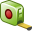# Measuring a Distance

The measure distance tool allows you to measure or validate the physical distance between two points in a model.

1. On the Tools tab, in the Tools group, click theMeasure distance icon.
2. Under Point1, use Ctrl+Shift+left click to snap to points (for example, named points, geometry points, geometry face centre, geometry edge centre, mesh vertices and grid).
3. Repeat Step 2 for Point 2.
The total distance, as well as the individual X axis, Y axis and Z axis distances, are displayed in the Distance (D), X distance, Y distance and Z distance fields respectively.
4. Click Close to close the dialog.